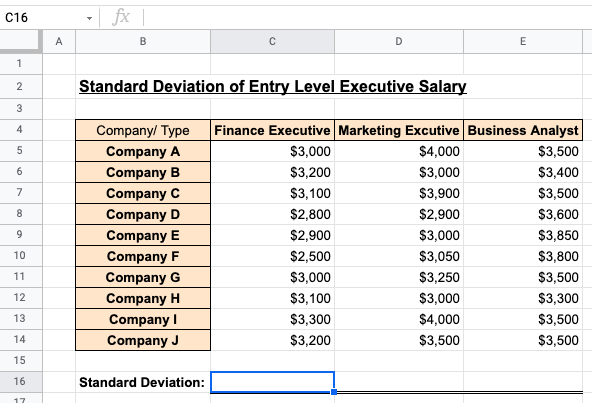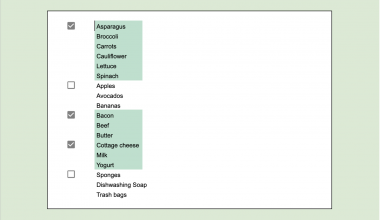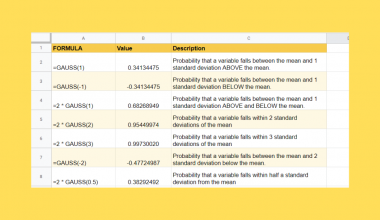# How to Use STDEVA Function in Google Sheets

Learning how to use the STDEVA function in Google Sheets is useful to find the standard deviation among an array of samples

Standard deviation is a tool to measure how spread out a group of data is to the average. A low standard deviation means the group of data is close to the average. However, a high standard deviation means the data group is further from the average and is more spread out.

Average in this context signifies the mean of the data. Mean is to measure the middle-point of a set of data. Usually, a set of data’s mean and standard deviation will be stated together. This will help us determine how closely or loosely the data is spread out.

For example, real estate agents assess the standard deviation of house prices in a given location so that they can tell their clients what kind of price fluctuation to expect.

Another example will be using standard deviation to predict the weather. If temperatures don’t change much from one day to the next, this results in a lower standard deviation. Hence, it allows the weather forecaster to accurately and confidently predict the weather on any given day.

## Anatomy of the STDEVA Function

The syntax of the `STDEVA` function is as follow:

`= STDEVA(value1, value2, …)`

Let us look at each term in the formula to understand what they mean:

• `=` is the equal sign which is how we start any function in Google Sheets.
• `STDEVA()` is our STDEVA function. It measures the standard deviation of a dataset.
• `value1` is the first value of a group of data
• `value2, …` are the rest of the values that you want to include

Here are some pointers you should take note of for the STDEVA function:

1. The STDEVA function will only work when two or more values are included in the formula. If only one value is included, the formula will return a #DIV/0 error if only one value is included.
2. STDEVA function will convert all text values to zero for calculation.
3. If you insert ‘False’ as a value in the formula, the STDEVA function will convert it to zero.
4. If you insert ‘True’ as a value in the formula, the STDEVA function will convert it to one.

## A Real-Life Example of Using the STDEVA Function

Let’s look at a real-life example of using the STDEVA function in Google Sheets.

In this example, imagine yourself as a human resource executive. One of the human resource department struggles is to figure out that is the correct amount of salary to offer to new hires.

There are many departments in a company, so it is hard to understand and gauge the industry standard for all departments. To stay relevant and competitive, you are tasked to find the standard deviation of each field’s salary.

Calculating the standard deviation of each industry will help determine the variation of salary in a certain field. This can easily be executed using the STDEVA function in Google Sheets.

You may make a copy of the spreadsheet using the link I have attached below.

Once you are ready and understand the concept of standard deviation, we can jump into writing the formula together!

## How to Use the STDEVA Function in Google Sheets

This section will go through each step needed to use the STDEVA function in Google Sheets. This guide will show you how we can get the standard deviation of the dataset seen previously.

Follow these steps to start using the STDEVA function:

1. First, let us select the cell that will contain our STDEVA function result. In this example, we will select C16 to insert the formula in.1. Next, we simply type the equal sign `=` to begin the function, followed by `STDEVA(`.1. You may find a pop-up box with a description of using the STDEVA function. We can click on the arrow located on the top-right-hand corner of the box to minimize it.1. Next, we will type in our arguments. We will select the salary range for a finance executive, which is C5:C14. Don’t forget to close the formula with a closing bracket `)`.1. Once we press Enter, the standard deviation is calculated to be 233.1. Now we can copy the same formula to D17 and D18 to calculate the standard deviation for a marketing executive and business analyst’s salary.As shown in the standard deviations, a marketing executive’s salary is the most volatile among these three industries as it has the highest standard deviation.

Hence, you now know that the pay of a marketing executive is highly negotiable and dependable on the prospect’s and hiring manager’s demands.

There you go! Now you can easily calculate and find the standard deviation for a set of data. This function can be applied to many more real-life scenarios and will come in handy!

Don’t forget to check out other cool functions in Google Sheets to enhance and simplify work for your everyday use!

Make sure to subscribe to our newsletter to be the first to know about the latest guides and tutorials from us.Our goal this year is to create lots of rich, bite-sized tutorials for Google Sheets users like you. If you liked this one, you'd love what we are working on! Readers receive ✨ early access ✨ to new content.

##### You May Also Like## How to Make an Organization Chart in Google Sheets (Org Chart)

Learning how to make an organization chart in Google Sheets is useful for showing the hierarchy of employees…## VLOOKUP with IMPORTRANGE in Google Sheets

This guide will explain how you can use a lookup table found on another worksheet using VLOOKUP and…## How to Highlight Multiple Groups and Control Checkboxes in Google Sheets

Highlighting multiple groups using checkboxes in your Google Sheets document makes it easier to read separate groups in…## How to Use RIGHT Function in Google Sheets

The RIGHT function in Google Sheets is useful to return a substring from the end of a string.…